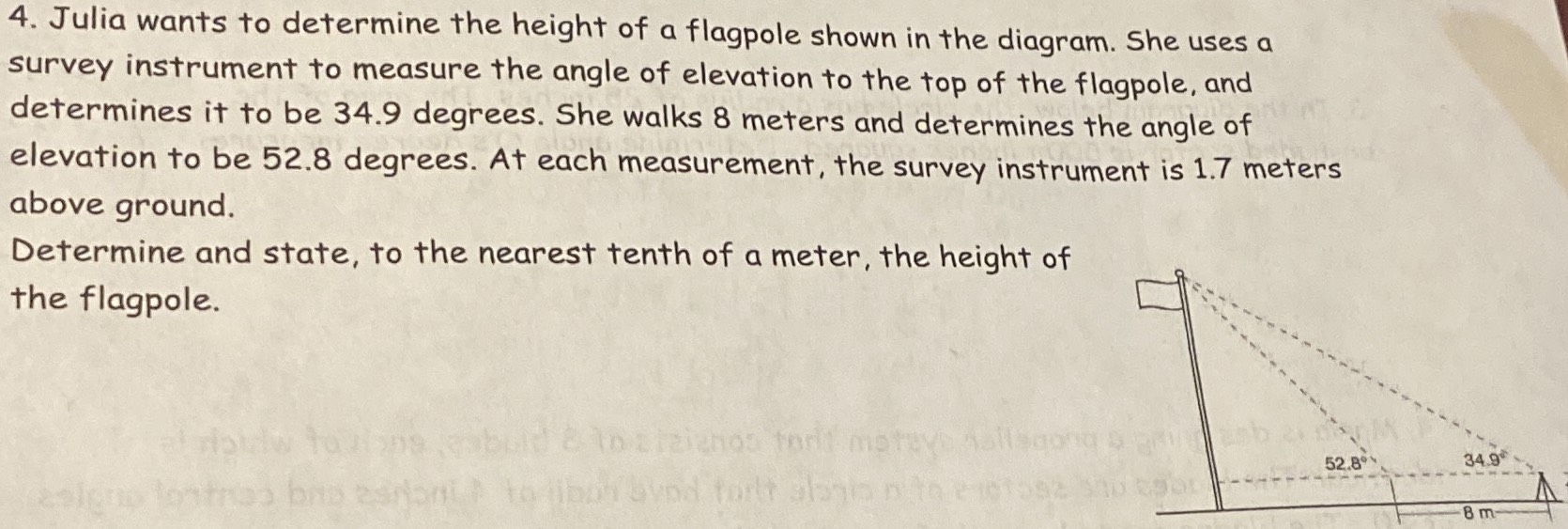### Still have math questions?

Trigonometry
Question4. Julia wants to determine the height of a flagpole shown in the diagram. She uses a survey instrument to measure the angle of elevation to the top of the flagpole, and determines it to be $$34.9$$ degrees. She walks $$8$$ meters and determines the angle of elevation to be $$52.8$$ degrees. At each measurement, the survey instrument is $$1.7$$ meters above ground. Determine and state, to the nearest tenth of a meter, the height of the flagpole.